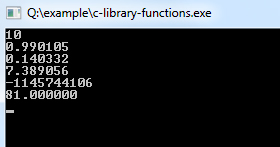## C Programming Tutorial Index

C library functions are provided by the system and stored in the library. In C programming, C library functions are also called inbuilt functions.

To use Inbuilt Function in C, you must include their respective header files containing prototypes and data definitions.

### C Program to Demonstrate the Use of Library Functions

Example:

``````#include<stdio.h>
#include<ctype.h>
#include<math.h>

void main()
{
int i = -10, e = 2, d = 10; //Variables Defining and Assign values
double d1 = 3.0, d2 = 4.0;

printf("%d\n", abs(i));